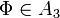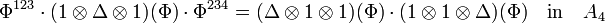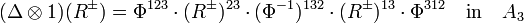# Associators with Frozen Feet

(diff) ← Older revision | Latest revision (diff) | Newer revision → (diff)

## The Goal

The purpose of the paperlet is to find an explicit formula for an associator with frozen feet. As I'm starting to write, I don't know such a formula. My hope is that as I type up all the relevant equations, a solution will emerge. I'll be just as happy if it emerges in somebody else's mind, provided (s)he shares her/his thoughts.

An associator is a solution$\Phi\in A_3$ of the pentagon equation and the hexagon equations (, , ):

 [Pentagon]$\Phi^{123}\cdot(1\otimes\Delta\otimes 1)(\Phi)\cdot\Phi^{234}=(\Delta\otimes 1\otimes 1)(\Phi)\cdot(1\otimes 1\otimes\Delta)(\Phi)\quad\mbox{in}\quad A_4$,

 [Hexagons]$(\Delta\otimes 1)(R^{\pm}) = \Phi^{123}\cdot (R^{\pm})^{23}\cdot(\Phi^{-1})^{132}\cdot(R^{\pm})^{13}\cdot\Phi^{312}\quad\mbox{in}\quad A_3$.